# Multiplying Rational Numbers Lesson Plan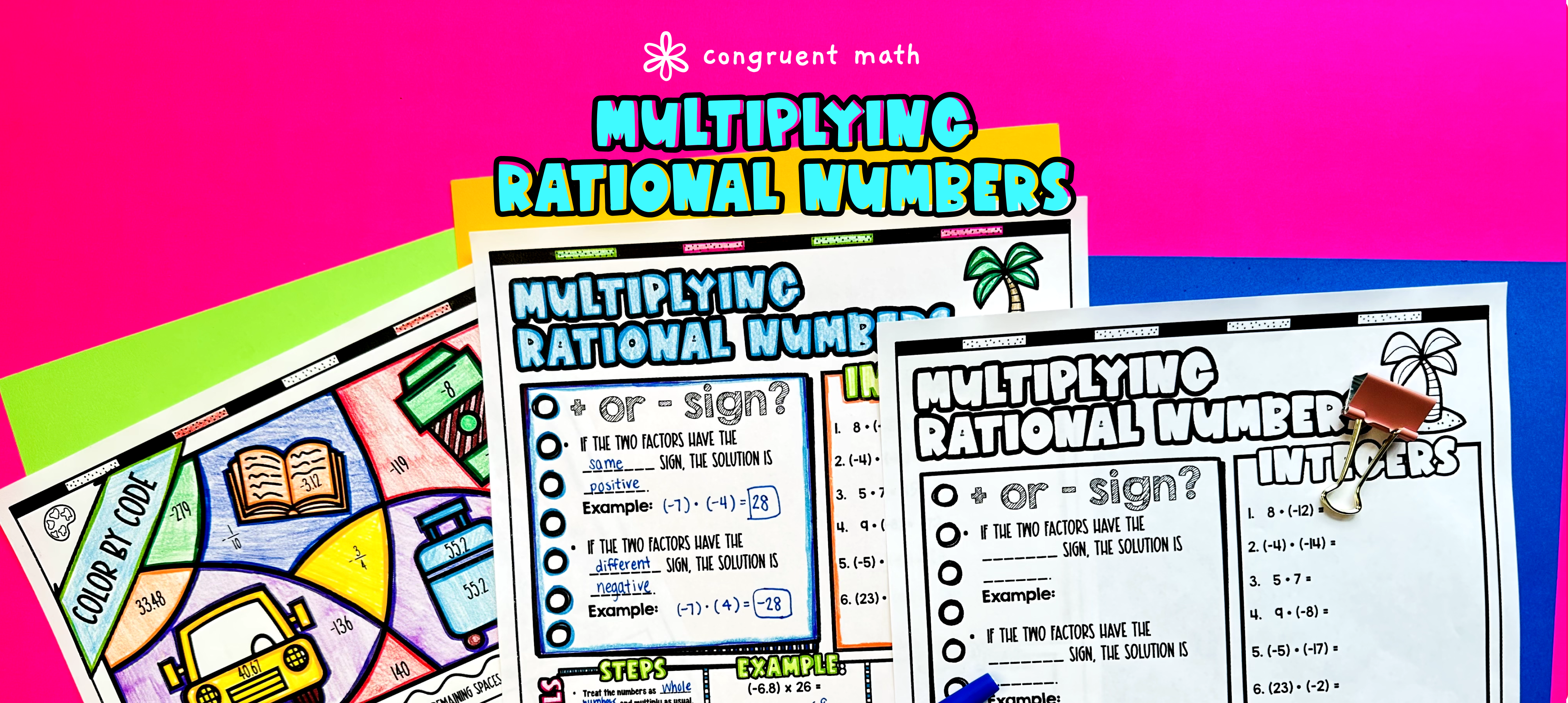Pin This

## Overview

Ever wondered how to teach multiplying rational numbers in an engaging way to your middle school students?

In this lesson plan, students will learn about multiplying fractions, integers, and decimals and their real-life applications. Through artistic, interactive guided notes, check for understanding, practice worksheets, and a color by number activity, students will gain a comprehensive understanding of multiplying rational numbers.

The lesson culminates with a real-life example that explores how multiplying rational numbers can be applied to picking a hotel when planning a trip.

## Get the Lesson Materials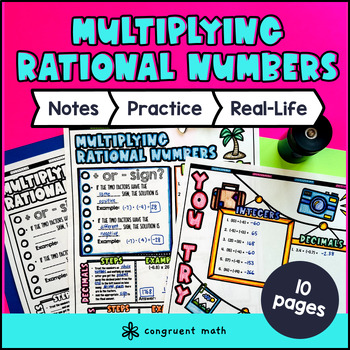\$4.25

## Learning Objectives

After this lesson, students will be able to:

• Multiply rational numbers including fractions, integers, and decimals

• Apply the concept of multiplication to solve real-life math problems involving rational numbers

## Prerequisites

Before this lesson, students should be familiar with:

• Basic multiplication skills and fluency in multiplying whole numbers

• Understanding of the concept of rational numbers and their representation on a number line

• Basic understanding of fractions, including how to multiply fractions

• Basic understanding of decimals and their relationship to fractions (optional, but helpful)

## Key Vocabulary

• Rational numbers

• Fractions

• Integers

• Decimals

• Multiply

## Procedure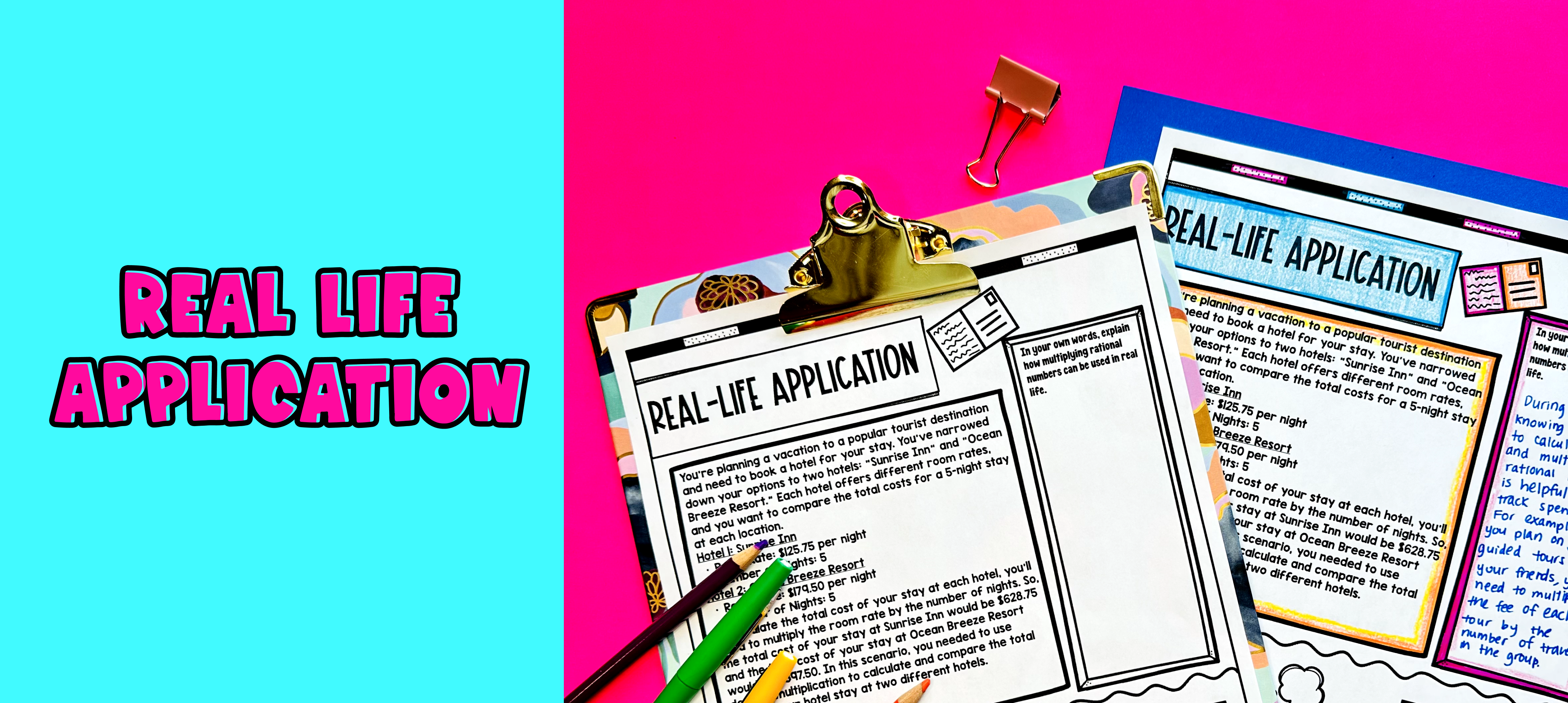Pin This

### Introduction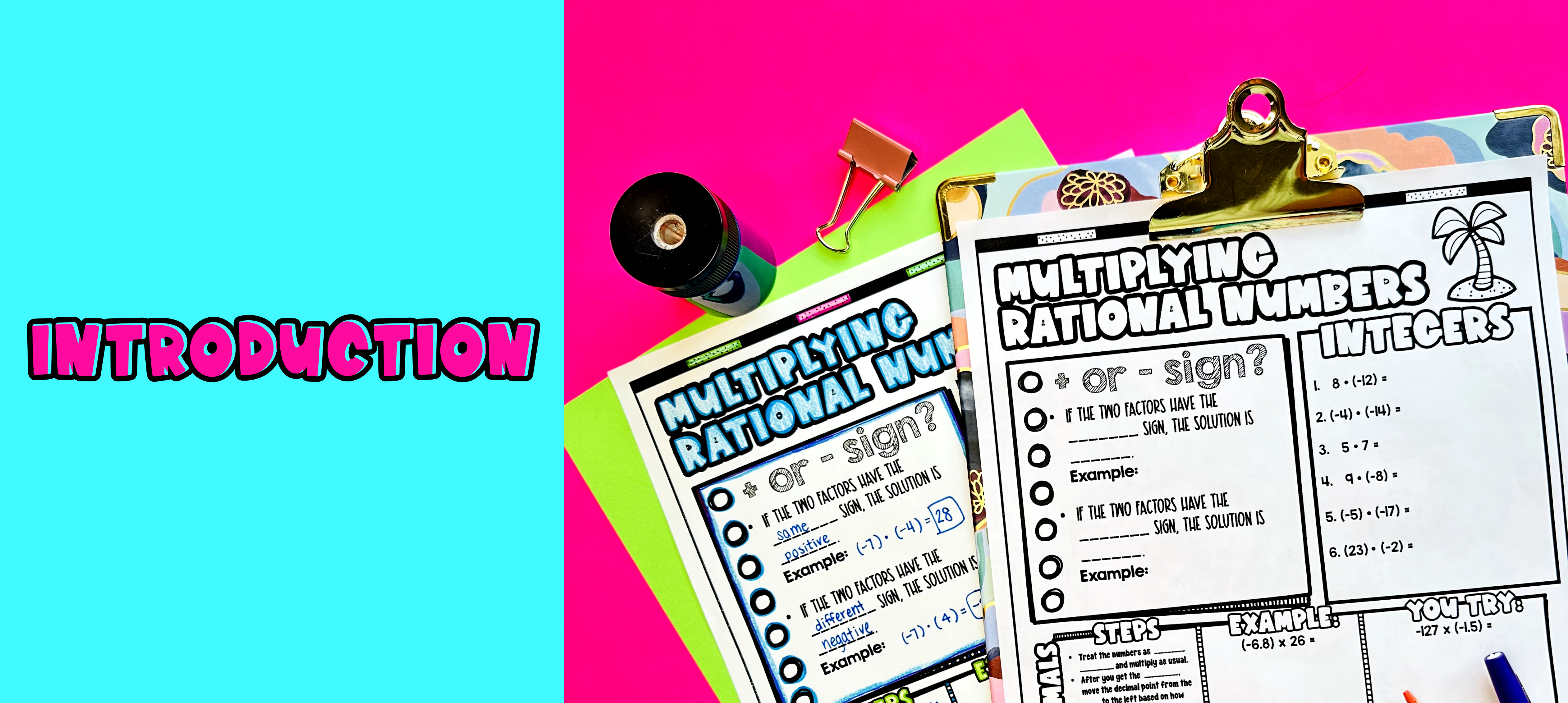Pin This

As a hook, ask students why understanding how to multiply rational numbers, including fractions, integers, and decimals, is important in real-life situations. Refer to the last page of the guided notes as well as the FAQs below for ideas.

Use the guided notes to introduce the concept of multiplying rational numbers. Walk through the key points of the topic, emphasizing the different strategies for multiplying fractions, integers, and decimals. Refer to the FAQ below for a walk through on this, as well as ideas on how to respond to common student questions.

• Check for Understanding: Have students walk through the "You Try!" section in the guided notes. Call on students to talk through their answers, potentially on the whiteboard or projector. Based on student responses, reteach concepts that students need extra help with.

Based on student responses, reteach concepts that students need extra help with. If your class has a wide range of proficiency levels, you can pull out students for reteaching and have more advanced students begin work on the practice exercises.

### Practice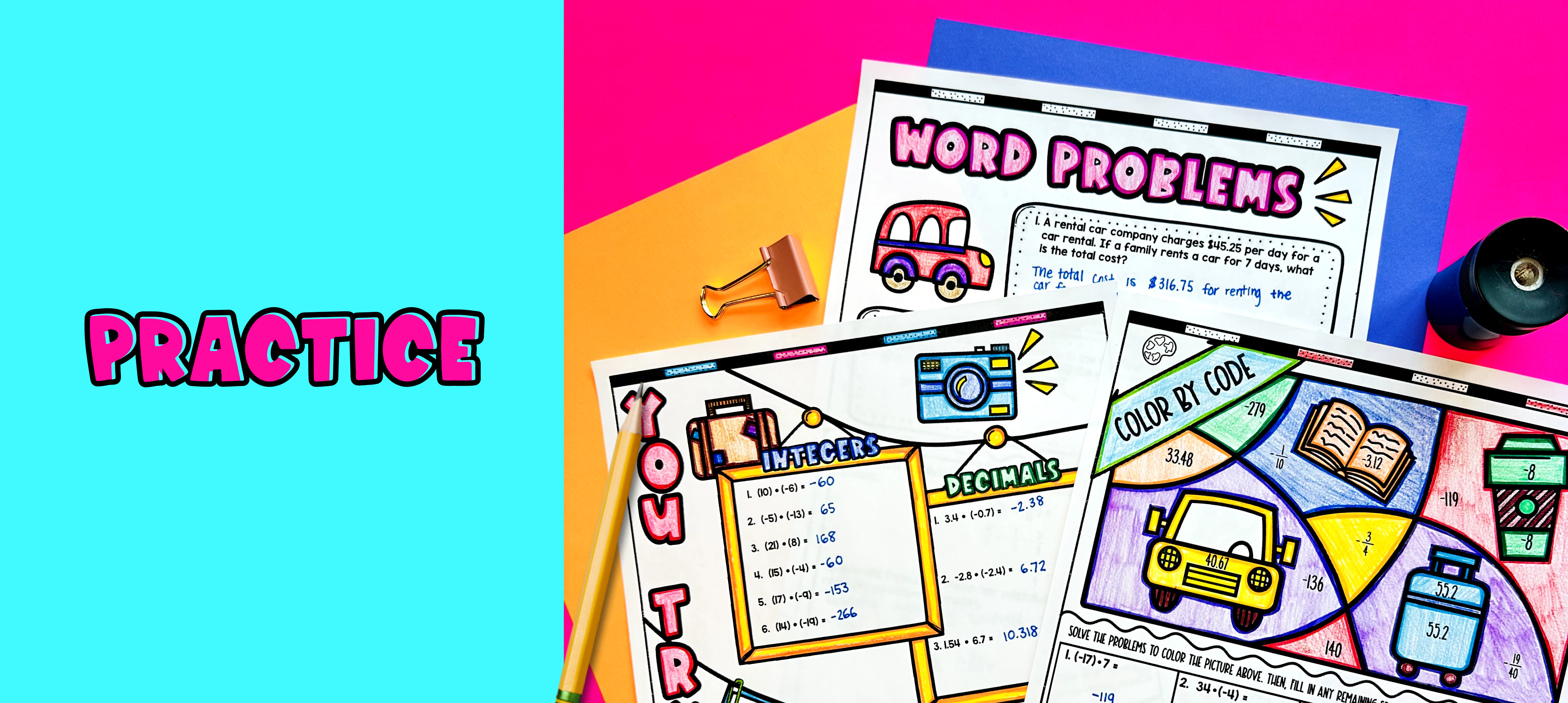Pin This

Have students practice multiplying rational numbers including fractions, integers, and decimals using the practice color by code activity provided in the resource. Walk around the classroom to answer any student questions and provide support as needed.

Fast finishers can dive into the word problems included in the resource for extra practice. You can also assign these activities as homework for the remainder of the class.

### Real-Life ApplicationPin This

Bring the class back together, and introduce the concept of rational number multiplication in real-life situations. Discuss with the students how multiplying rational numbers can be useful in various real-world scenarios, such as calculating distances, time, or money.

For example, explain to the students that when planning a road trip, they may need to calculate the total price of a hotel stay. In this case, they may encounter rational numbers, such as fractions or decimals, when multiplying the nightly rate by the number of nights. By understanding how to multiply rational numbers, they will be able to accurately determine the total price of the hotel stay.

Refer to the FAQ section of the resource for more ideas on how to further engage the students and explore different real-life applications of multiplying rational numbers.

## Extensions

If you’re looking for digital practice for multiplying rational numbers including fractions, integers, and decimals, try the Pixel Art activities in Google Sheets. Every answer is automatically checked, and correct answers unlock parts of a mystery picture. It’s incredibly fun, and a powerful tool for differentiation.

Here is an activity to try: